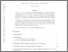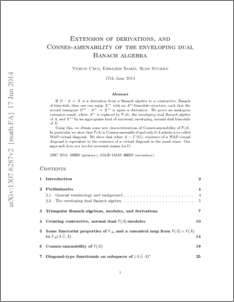# Extension of derivations, and Connes-amenability of the enveloping dual Banach algebra

Choi, Yemon and Samei, Ebrahim and Stokke, Ross (2015) Extension of derivations, and Connes-amenability of the enveloping dual Banach algebra. Mathematica Scandinavica, 117 (2). pp. 258-303. ISSN 0025-5521Preview
PDF (1307.6287v2)
1307.6287v2.pdf - Accepted Version
If $D:A \to X$ is a derivation from a Banach algebra to a contractive, Banach $A$-bimodule, then one can equip $X^{**}$ with an $A^{**}$-bimodule structure, such that the second transpose $D^{**}: A^{**} \to X^{**}$ is again a derivation. We prove an analogous extension result, where $A^{**}$ is replaced by $\F(A)$, the \emph{enveloping dual Banach algebra} of $A$, and $X^{**}$ by an appropriate kind of universal, enveloping, normal dual bimodule of $X$. Using this, we obtain some new characterizations of Connes-amenability of $\F(A)$. In particular we show that $\F(A)$ is Connes-amenable if and only if $A$ admits a so-called WAP-virtual diagonal. We show that when $A=L^1(G)$, existence of a WAP-virtual diagonal is equivalent to the existence of a virtual diagonal in the usual sense. Our approach does not involve invariant means for $G$.View Item